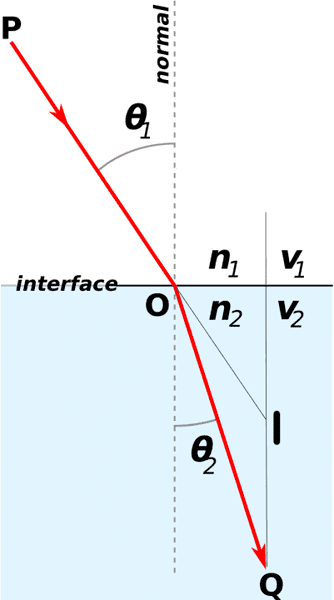# Apparent Depth Equation

• B
• aeri

#### aeri

Hi, can someone please explain to me where this equation derives from and how it works?
d'=d(n2/n1)
where d'=the apparent depth of the object, d=the actual depth, n1= the index of refraction of the medium in which the refracted light travels, and n1= the index of refraction of the medium in which the incident light travels

Hi, can someone please explain to me where this equation derives from and how it works?
d'=d(n2/n1)
where d'=the apparent depth of the object, d=the actual depth, n1= the index of refraction of the medium in which the refracted light travels, and n1= the index of refraction of the medium in which the incident light travels
Welcome to the PF.

Have you learned about Snell's Law yet?https://en.wikipedia.org/wiki/Snell's_law

Welcome to the PF.

Have you learned about Snell's Law yet?https://en.wikipedia.org/wiki/Snell's_law
Yes:)

Yes:)
Great! Then you should be able to make a first attempt at deriving that depth equation. Start with the first figure at the wikipedia link, and change it to be an observer looking down at an object under water...

Great! Then you should be able to make a first attempt at deriving that depth equation. Start with the first figure at the wikipedia link, and change it to be an observer looking down at an object under water...
Something like this? Where P is where the observer is and I is the image of where the object appears to be•berkeman